# Implicit Functions

Also found in: Dictionary.
Related to Implicit Functions: implicit differentiation
The following article is from The Great Soviet Encyclopedia (1979). It might be outdated or ideologically biased.

## Implicit Functions

functions defined by relations between independent variables that have not been explicitly solved for the latter; such relations are one of the methods of defining functions. For example, the relation

x2 + y2 − 1 = 0

defines an implicit function

y = y (x)

and the relations

x = ρ cos φ sin φ

x = ρ sin φ sin θ, z = ρ cos θ

define implicit functions

ρ = ρ(x, y, z) φ = φ(x, y, z) θ = θ(x, y, z)

In the simplest cases, the relations defining implicit functions may be solved in terms of elementary functions, that is, elementary functions may be found that satisfy these relations. Thus, in the first example above we have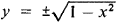and in the second,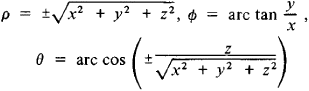However, in general, such elementary functions cannot be found. Implicit functions may be single-valued or many-valued. Not every relation (or system of relations) between variables defines an implicit function. Thus, if the values of the variables are restricted to real numbers, then the relation x2 + y2 + 1 = 0 does not define an implicit function, since the function exy = 0 is not satisfied by a single pair of real or complex numbers ξ and y; the relation e”y = 0 is not satisfied by a single pair of real or complex numbers ξ and y. The existence theorem for implicit functions in its simplest form asserts that if a function F (x, y) vanishes for a pair of values x = x0, and y = y0 [F (x0, y0 ≠ 0] and is differentiable in a neighborhood of the point (x0, y0 and, moreover, Fx(x, y) and Fy(x, y) are continuous in this neighborhood and Fy(x0, y0) ≠ 0, then in a sufficiently small neighborhood of the point x0 there exists a unique single-valued continuous function y = y (x) that satisfies the relation F (x, y) = 0 and takes on the value y0 for x = x0; also, y’(x) = —Fx(x, y)/Fy(x, y).

Power series are often used for the approximate calculation of values of an implicit function near x0, where the function’s value y0 is known. Thus, if F (x, y) is an analytic function—that is, it may be expanded as a convergent double power series in the neighborhood of the point (x0, y0)]—and if Fy(x0, y0) ≠ 0, then the implicit function defined by the relation F (x, y) = 0 may be obtained in the form of a power series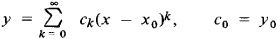which is convergent in a certain neighborhood of the point x = x0 The coefficients Ck, k = 1, 2, . . . , may be found either by substituting this series in the relation F (x, y) = 0 or by successively differentiating this relation with respect to x. For example, if the implicit function is given by the relation

y5 + xy − 1 = 0, x0 = 0, y0 = 1

then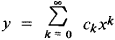and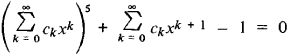from which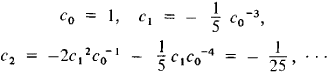If the relation F (x, y) = 0 may be put in the form y = a + xφ(y), where φ(y) is an analytic function, then the implicit function that is defined by this relation and that assumes the value a at x = 0 can be expanded in a Lagrange series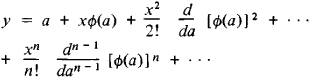which is convergent in a certain neighborhood of the point x = 0. For example, the relation y = a + x sin y (Kepler’s equation) vields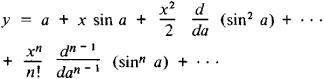In the general case, the values of an implicit function may be calculated by the method of successive approximations.

### REFERENCES

Smirnov, V. I. Kurs vysshei matematiki, vol. 1, 22nd ed. Moscow, 1967. Vol. 3, part 2, 8th ed. Moscow, 1969.
Fikhtengol’ts, G. M. Kurs differentsial’nogo i integral’nogo ischisleniia, 7th ed., vol. 1. Moscow, 1969.
Kudriavtsev, L. D. Matematicheskii analiz, vol. 2. Moscow, 1970.
References in periodicals archive ?
Recently this method of variable projection has been extended in a restricted sense  in the field of inverse problems, which is not related to our method of either modeling or optimization, nor is the methodology related to the implicit function properties.
The above follows from the implicit function theorem (IFT) [3, Theorem 13.7, page 374] where k [member of] [K.sub.0] is the independent variable for the existence of the P(k) function.
The above results for the functions [h.sub.j](P, k) = 0 (j = 1, 2, ..., [N.sub.p]) having a unique implicit function of k, denoted P(k) by the IFT [3, Theorem 13.7, page 374], require that det [parallel] [partial derivative][h.sub.i](P,k)/[partial derivative][P.sub.j] [parallel] = det [parallel] [partial derivative][Q.sub.T]/[partial derivative][P.sub.j][partial derivative][P.sub.i] [parallel] [not equal to] 0 on an open set S, k [member of] S.
In practical situations, particularly in many exploration applications, the implicit functions of obstacles to be modeled are not available.
Once the multiscale implicit functions for each subdomain are constructed, function values at arbitrary position in overall domain can be evaluated by partition of unity method that calculates a reconstructed function value by weighted sum of local functions for neighbor subdomains.
1: procedure MLTSCL_KRNL_SRFC({([[??].sub.i], [f.sub.I])}) 2: m [left arrow] Decompose the domain [omega] 3: for k [right arrow] 1, m do 4: Build up the linear system for [f.sub.k] (Equation (3)) 5: Solve the linear system (Equation (3)) 6: Calculate the coefficients (Equation (4)) 7: F [right arrow] F + [f.sub.k] 9: end for 10: Ray-trace or polygonise F with LOD 11: end procedure 3.2 Calculating Implicit Functions
etc., according to the known theorem of implicit functions (Litvin et al., 1986) det [G.sup.q] = 0, and the condition of correctness of the manifold description (5) is not carried out.
We also show that numerous contrastive conditions of the existing literature enjoy the format of our newly introduced implicit function besides admitting several new and natural contrastive conditions.
In this section, we introduce a new class of implicit function which is different from the one considered in Popa  and furnish examples to substantiate the worth of this definition.
If the improvement of the solution for an one-criterion sub-problem in the complex optimisation, using special mathematical tools for acceleration of the computing process GMRG, is called complex optimal correction of ESS of EPS, the improvement of the solution of multi-objective sub-problems of the complex optimisation using special mathematical tools for acceleration of the computing process [application instead of the gradient of the one implicit function the array of the multi-objective descent or rise (dependent on the minimisation or maximisation of the partners objective functions)], is called complex multiobjective or Pareto-optimal correction of ESS of IPS.
as an implicit function X(Y, D) of the array of independent variables (controls) Y and of the array of initial information D.
The equations W give thus the implicit function [phi], which determines the whole point z according to the vector x of independent coordinates of the point z in such a way that W ([phi](x)) = 0.

Site: Follow: Share:
Open / Close